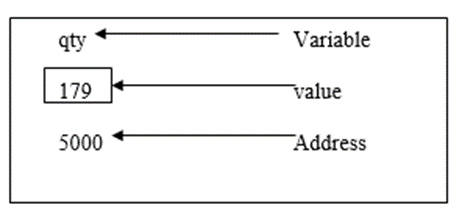# How to multiply two matrices using pointers in C?

Pointer is a variable that stores the address of another variable.

## Features of Pointers

• Pointer saves the memory space.
• The execution time of a pointer is faster because of the direct access to a memory location.
• With the help of pointers, the memory is accessed efficiently i.e. memory is allocated and deallocated dynamically.
• Pointers are used with data structures.

Pointer declaration, initialization and accessing

Consider the following statement −

int qty = 179;

In the memory, the variable can be represented as shown below −## Declaration

Declaring a pointer can be done as shown below −

Int *p;

It means ‘p’ is a pointer variable which holds the address of another integer variable.

## Initialization

The address operator (&) is used to initialize a pointer variable.

For example,

int qty = 175;
int *p;
p= &qty;

## Accessing a variable through its pointer

To access the value of the variable, indirection operator (*) is used.

## Example

Following is the C program to multiply the two matrices by using pointers −

Live Demo

#include <stdio.h>
#define ROW 3
#define COL 3
/* Function declarations */
void matrixInput(int mat[][COL]);
void matrixPrint(int mat[][COL]);
void matrixMultiply(int mat1[][COL], int mat2[][COL], int res[][COL]);
int main() {
int mat1[ROW][COL];
int mat2[ROW][COL];
int product[ROW][COL];
printf("Enter elements in first matrix of size %dx%d", ROW, COL);
matrixInput(mat1);
printf("Enter elements in second matrix of size %dx%d", ROW, COL);
matrixInput(mat2);
matrixMultiply(mat1, mat2, product);
printf("Product of both matrices is : ");
matrixPrint(product);
return 0;
}
void matrixInput(int mat[][COL]) {
int row, col;
for (row = 0; row < ROW; row++) {
for (col = 0; col < COL; col++) {
scanf("%d", (*(mat + row) + col));
}
}
}
void matrixPrint(int mat[][COL]) {
int row, col;
for (row = 0; row < ROW; row++) {
for (col = 0; col < COL; col++) {
printf("%d ", *(*(mat + row) + col));
}
printf("");
}
}
void matrixMultiply(int mat1[][COL], int mat2[][COL], int res[][COL]) {
int row, col, i;
int sum;
for (row = 0; row < ROW; row++) {
for (col = 0; col < COL; col++) {
sum = 0;
for (i = 0; i < COL; i++) {
sum += (*(*(mat1 + row) + i)) * (*(*(mat2 + i) + col));
}
*(*(res + row) + col) = sum;
}
}
}

## Output

When the above program is executed, it produces the following output −

Enter elements in first matrix of size 3x3
2 3 1
2 5 6
2 6 8
Enter elements in second matrix of size 3x3
1 2 1
2 3 4
5 6 7
Product of both matrices is :
13 19 21
42 55 64
54 70 82